Publicité

# Parallel Resonance Ckt in Network Theory.pptx

22 Mar 2023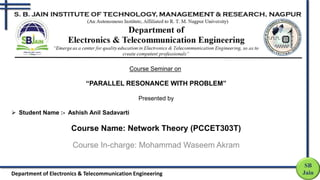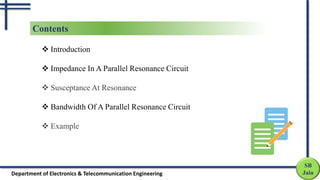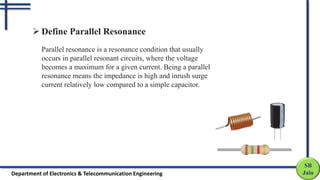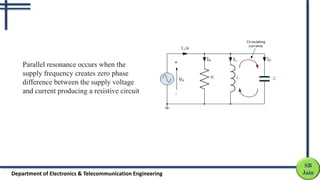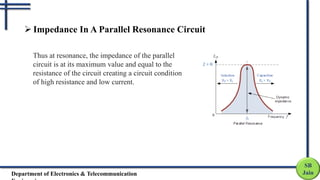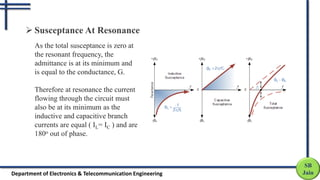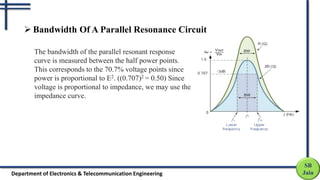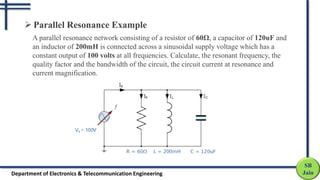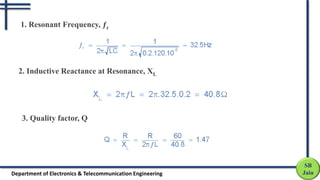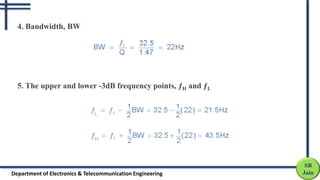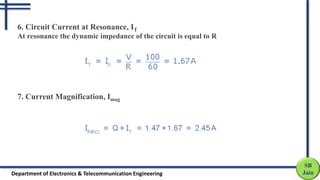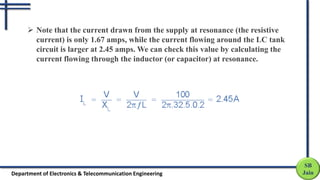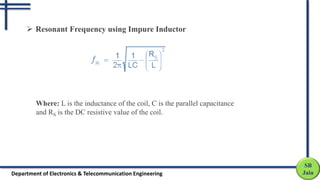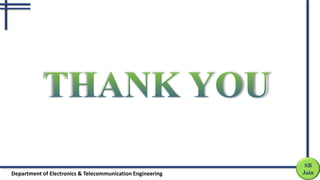1 sur 14
Publicité

### Parallel Resonance Ckt in Network Theory.pptx

1. Course Seminar on “PARALLEL RESONANCE WITH PROBLEM” Presented by  Student Name :- Ashish Anil Sadavarti Course Name: Network Theory (PCCET303T) Course In-charge: Mohammad Waseem Akram SB Jain Department of Electronics & Telecommunication Engineering
2. SB Jain Department of Electronics & Telecommunication Engineering Contents  Introduction  Impedance In A Parallel Resonance Circuit  Susceptance At Resonance  Bandwidth Of A Parallel Resonance Circuit  Example
3. SB Jain Department of Electronics & Telecommunication Engineering  Define Parallel Resonance Parallel resonance is a resonance condition that usually occurs in parallel resonant circuits, where the voltage becomes a maximum for a given current. Being a parallel resonance means the impedance is high and inrush surge current relatively low compared to a simple capacitor.
4. SB Jain Department of Electronics & Telecommunication Engineering Parallel resonance occurs when the supply frequency creates zero phase difference between the supply voltage and current producing a resistive circuit
5. SB Jain Department of Electronics & Telecommunication  Impedance In A Parallel Resonance Circuit Thus at resonance, the impedance of the parallel circuit is at its maximum value and equal to the resistance of the circuit creating a circuit condition of high resistance and low current.
6. SB Jain Department of Electronics & Telecommunication Engineering  Susceptance At Resonance As the total susceptance is zero at the resonant frequency, the admittance is at its minimum and is equal to the conductance, G. Therefore at resonance the current flowing through the circuit must also be at its minimum as the inductive and capacitive branch currents are equal ( IL= IC ) and are 180o out of phase.
7. SB Jain Department of Electronics & Telecommunication Engineering The bandwidth of the parallel resonant response curve is measured between the half power points. This corresponds to the 70.7% voltage points since power is proportional to E2. ((0.707)2 = 0.50) Since voltage is proportional to impedance, we may use the impedance curve.  Bandwidth Of A Parallel Resonance Circuit
8. SB Jain Department of Electronics & Telecommunication Engineering A parallel resonance network consisting of a resistor of 60Ω, a capacitor of 120uF and an inductor of 200mH is connected across a sinusoidal supply voltage which has a constant output of 100 volts at all frequencies. Calculate, the resonant frequency, the quality factor and the bandwidth of the circuit, the circuit current at resonance and current magnification.  Parallel Resonance Example
9. SB Jain Department of Electronics & Telecommunication Engineering 2. Inductive Reactance at Resonance, XL 3. Quality factor, Q 1. Resonant Frequency, ƒr
10. SB Jain Department of Electronics & Telecommunication Engineering 4. Bandwidth, BW 5. The upper and lower -3dB frequency points, ƒH and ƒL
11. SB Jain Department of Electronics & Telecommunication Engineering 6. Circuit Current at Resonance, IT At resonance the dynamic impedance of the circuit is equal to R 7. Current Magnification, Imag
12. SB Jain Department of Electronics & Telecommunication Engineering  Note that the current drawn from the supply at resonance (the resistive current) is only 1.67 amps, while the current flowing around the LC tank circuit is larger at 2.45 amps. We can check this value by calculating the current flowing through the inductor (or capacitor) at resonance.
13. SB Jain Department of Electronics & Telecommunication Engineering  Resonant Frequency using Impure Inductor Where: L is the inductance of the coil, C is the parallel capacitance and RS is the DC resistive value of the coil.
14. SB Jain Department of Electronics & Telecommunication Engineering
Publicité